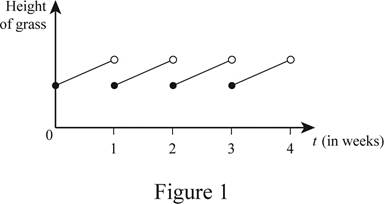# A rough graph of the height of the grass as a function of time over the period of four weeks.### Single Variable Calculus: Concepts...

4th Edition
James Stewart
Publisher: Cengage Learning
ISBN: 9781337687805### Single Variable Calculus: Concepts...

4th Edition
James Stewart
Publisher: Cengage Learning
ISBN: 9781337687805

#### Solutions

Chapter 1.1, Problem 19E
To determine

## To sketch: A rough graph of the height of the grass as a function of time over the period of four weeks.

Expert Solution

### Explanation of Solution

Let the time over four hour-week period (four Wednesdays) represented in the x-axis and the height of the grass over the week represented in the y-axis.

Generally, as days increases the length of the grass increases. Thus, the graph of the height of the grass as a function of time over the course of a four hour-week period is shown below in Figure 1.In Figure 1, the open dot indicates that the point is excluded and the closed dot indicates that the point is included.

Note: The height of the grass for every week is not necessary to be same in length. So, the graph may vary depends on the grass height.

### Have a homework question?

Subscribe to bartleby learn! Ask subject matter experts 30 homework questions each month. Plus, you’ll have access to millions of step-by-step textbook answers!